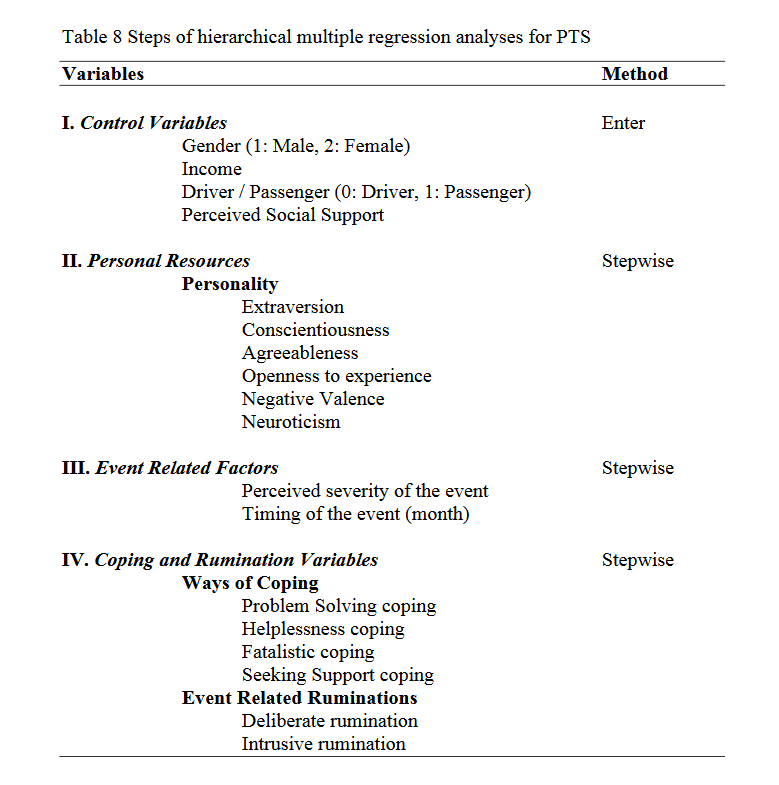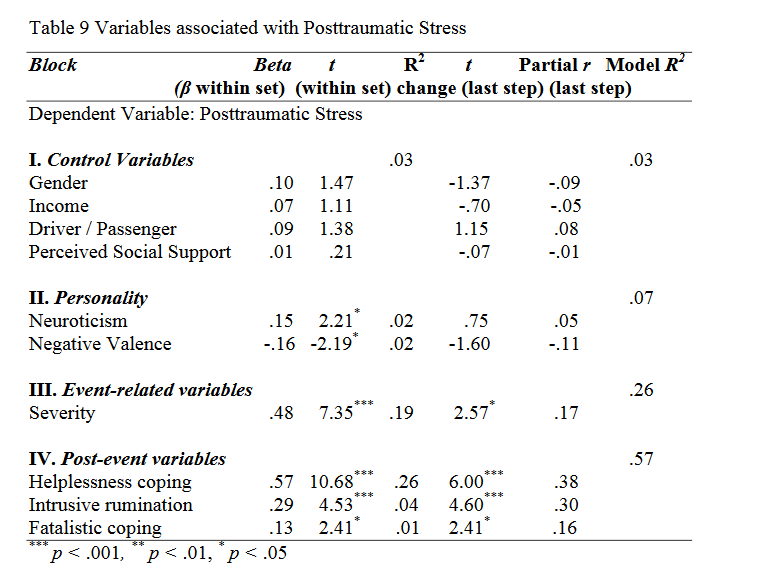Call Choice Point Psychological today: 587-362-0640

In the present study, a set of hierarchical multiple regression analyses were conducted for both dependent variables of the study (posttraumatic growth and posttraumatic stress) and all of their subscales. The aim of these analyses was to examine the effects of personality traits, event related factors, variables related with coping and rumination process on PTG and PTS, by controlling the effects of sociodemographic characteristics correlated with PTS and PTG (gender and income), perceived social support, and the role of the participants during the accident (driver or passenger).

## Predictors of PTS

A hierarchical multiple regression analysis was performed in order to examine the predictor variables of posttraumatic stress and to test the Hypothesis 1 (After controlling for the effect of gender, income, being driver or passenger during the accident, and the perceived social support; the perceived severity of the accident, coping, and rumination will predict (i.e. explain a significant variance) PTS scores and all of the three domains) of the present study. As can be seen from Table 8, the variables were entered into the regression equation in four steps. In order to control for the effects of gender, income, perceived social support, and the role of the participants during the accident (driver or passenger), these variables were forced to ‘enter’ into the equation in the first step. In the second, third, and fourth steps of the regression, stepwise method was used with the aim of revealing significant associates of PTS. In the second step of the regression, the personality variables (extraversion, conscientiousness, agreeableness, openness to experience, negative valence, and neuroticism) were entered into the equation. In the third step, event related factors (timing and perceived severity of the event) were added to the equation. Finally, in the last step, coping and rumination variables (problem solving, helplessness, seeking support, and fatalistic coping strategies; deliberate and intrusive ruminations) were entered to the equation.As can be seen from Table 9, the findings of the hierarchical regression analysis conducted for PTS showed that 3% of the variance was explained by control variables of the study. None of the control variables was significant.

From the personality variables, neuroticism (β = .15, t = 2.21, p < .05) positively predicted PTS, whereas, negative valence (β = .16, t = 2.19, p < .05) was negatively related to the outcome variable. The entrance of neuroticism into the equation increased the explained variance to 5% (R2 change = .02, F change (1, 219) = 4.86, p < .05), and with the inclusion of negative valence to the 54 equation, the explained variance reached 7% (R2 change = .02, F change (1, 218) = 4.79, p < .05).

The entrance of event related variables into the equation improved the explained variance to 26% (R2 change = .19, F change (1, 217) = 53.99, p < .001). Only perceived severity of the event positively predicted PTS (β = .48, t = 7.35, p < .001).

From coping and rumination variables, helplessness coping positively predicted PTS (β = .57, t = 10.68, p < .001), and its inclusion to the equation increased the explained variance to 52% (R2 change = .26, F change (1, 216) = 114.16, p < .001). Furthermore, intrusive rumination (β = .29, t = 4.53, p < .001) positively predicted PTS, and its entrance into the equation increased the explained variance to 56% (R2 change = .04, F change (1, 215) = 20.50, p < .001). Lastly, fatalistic coping (β = .13, t = 2.41, p < .05) positively predicted PTS and ameliorated the explained variance to 57% (R2 change = .01, F change (1, 214) = 5.78, p < .05).

With all the variables in the equation, in the last step, perceived severity of the event (t = 2.57, p < .01), helplessness coping (t = 6.00, p < .001), intrusive rumination (t = 4.60, p < .001), and fatalistic coping (t = 2.41, p < .01) remained to be significant predictors of PTS.